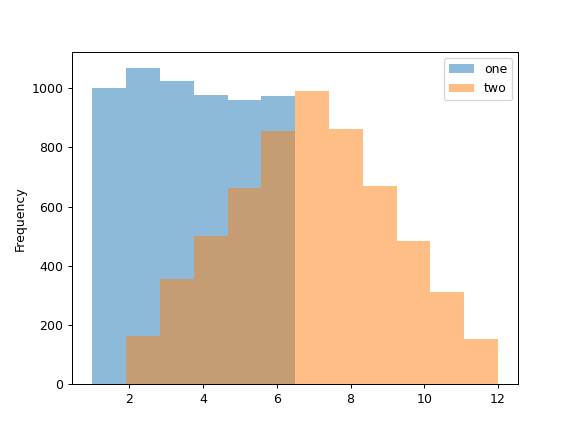# pandas.Series.plot.hist¶

Series.plot.hist(by=None, bins=10, **kwargs)[source]

Draw one histogram of the DataFrame’s columns.

A histogram is a representation of the distribution of data. This function groups the values of all given Series in the DataFrame into bins and draws all bins in one matplotlib.axes.Axes. This is useful when the DataFrame’s Series are in a similar scale.

Parameters
bystr or sequence, optional

Column in the DataFrame to group by.

binsint, default 10

Number of histogram bins to be used.

**kwargs

Additional keyword arguments are documented in DataFrame.plot().

Returns
class:matplotlib.AxesSubplot

Return a histogram plot.

DataFrame.hist

Draw histograms per DataFrame’s Series.

Series.hist

Draw a histogram with Series’ data.

Examples

When we draw a dice 6000 times, we expect to get each value around 1000 times. But when we draw two dices and sum the result, the distribution is going to be quite different. A histogram illustrates those distributions.

>>> df = pd.DataFrame(
...     np.random.randint(1, 7, 6000),
...     columns = ['one'])
>>> df['two'] = df['one'] + np.random.randint(1, 7, 6000)
>>> ax = df.plot.hist(bins=12, alpha=0.5)